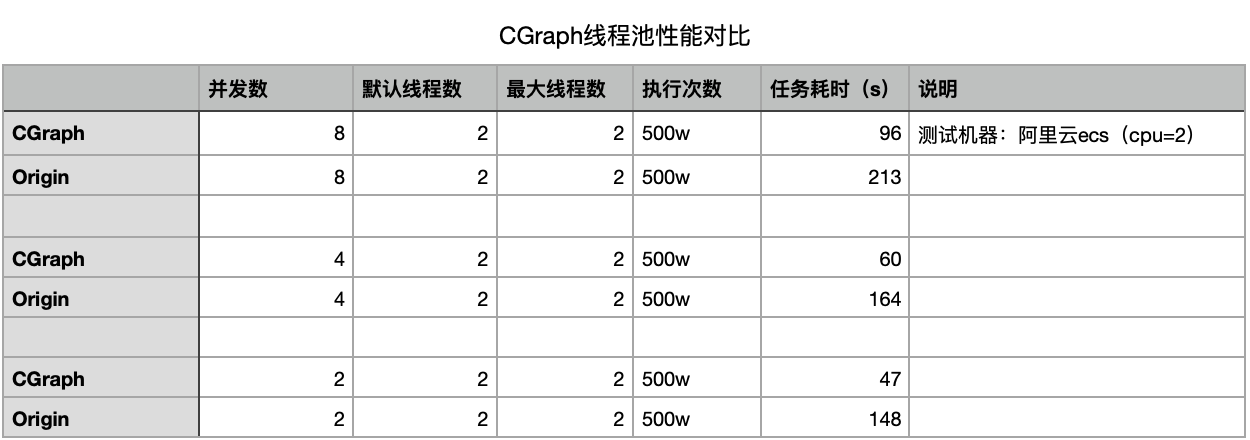## 使用Demo

``````int add(int i, int j) {
return i + j;
}

static float minusBy5(float i) {
return i - 5.0f;
}

class myFunction {
public:
std::string pow2(std::string& str) const {
int result = 1;
int pow = power_;
while (pow--) {
result *= (int)atoi(str.c_str());
}
return "multiply result is : " + std::to_string(result);
}

static int divide(int i, int j) {
if (0 == j) {
return 0;
}

return i / j;
}

int power_ = 2;
};

``````

``````void tutorial_threadpool() {

int i = 6, j = 3;
std::string str = "5";
myFunction mf;

/**
* 可以通过lambda表达式传递函数
* 也可以传入任意多个参数
* 方法返回值也可以是任意类型
*/
auto r1 = tp->commit([i, j] { return add(i, j); });
auto r2 = tp->commit(std::bind(minusBy5, 8.5f));
auto r3 = tp->commit(std::bind(&myFunction::pow2, mf, str));
std::future<int> r4 = tp->commit(std::bind(&myFunction::divide, i, j));    // commit()返回值，实际上是一个std::future<T>类型

/**
* 返回值可以是int、string等各种类型
* 调用get()方法，表示阻塞等待改函数执行完毕
* 不调用get()方法，表示不阻塞等待
*/
std::cout << r1.get() << std::endl;
// std::cout << r2.get() << std::endl;    // 不阻塞等待该函数执行完毕
std::cout << r3.get() << std::endl;
std::cout << r4.get() << std::endl;
}
``````## 性能对比``````#include <iostream>
#include <omp.h>
#include <tbb/tbb.h>

void myPrint(int x) {
std::cout << x << std::endl;
}

void openmp_version() {
/* openmp版本，并发打印0~99 */
for(int i = 0; i < 100; i++) {
myPrint(i);
}
}

void tbb_version()
{
/* tbb版本，并发打印0~99 */
tbb::parallel_for(0, 100, 1, myPrint);
}

void cgraph_version() {
/* CGraph版本，并发打印0~99 */
for (int i = 0; i < 100; i++) {
tp->commit([i] { myPrint(i); });
}
}

int main() {
openmp_version();
tbb_version();
cgraph_version();
return 0;
}
``````

## TODO

cas机制## 本章小结``````    					[2021.09.04 by Chunel]
``````

#### 个人信息

github地址: https://github.com/ChunelFeng